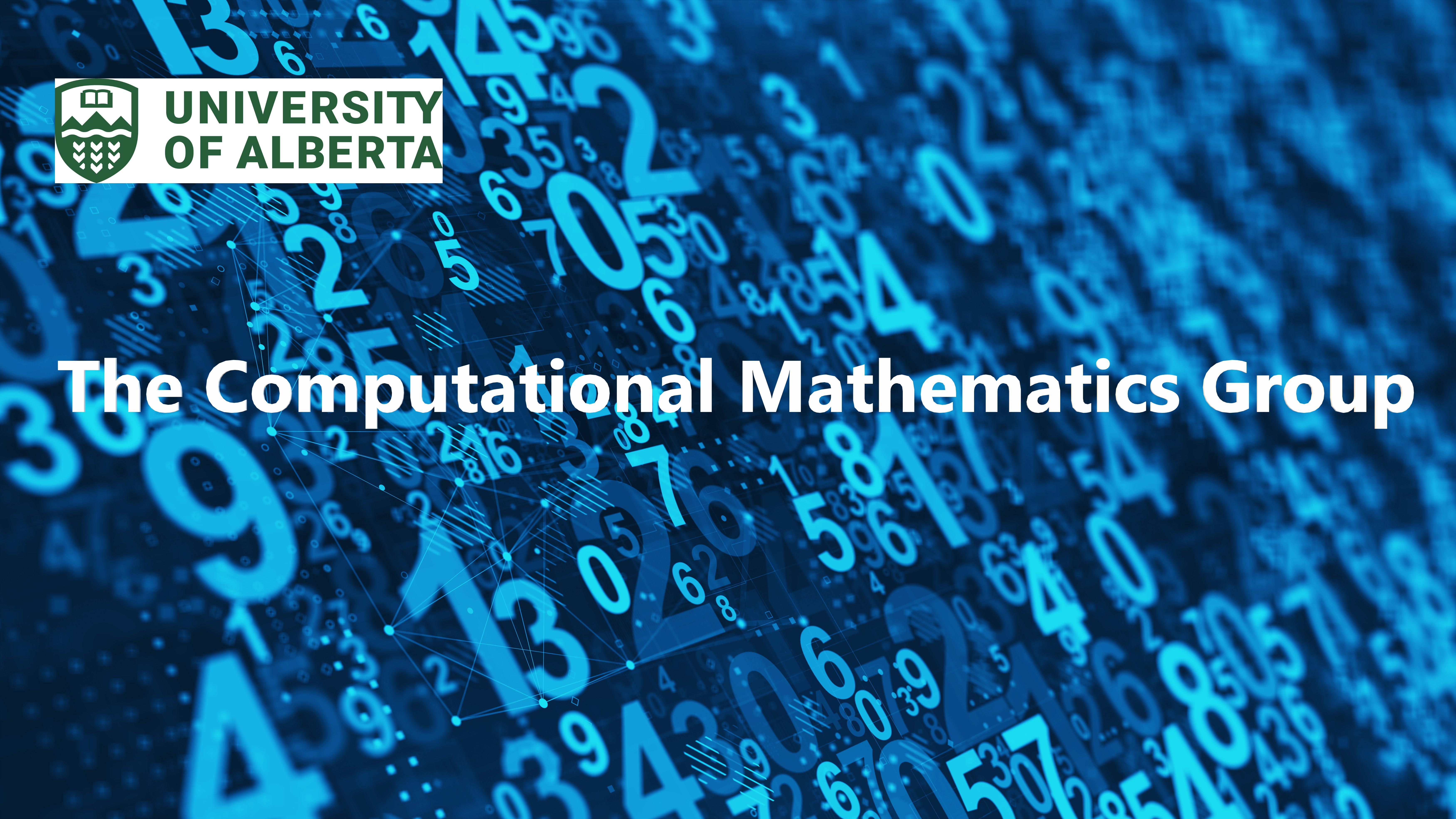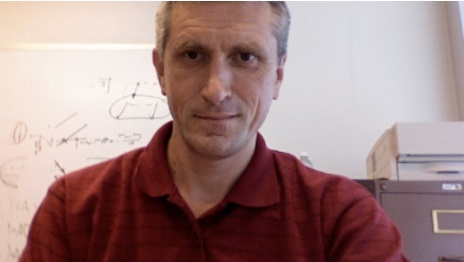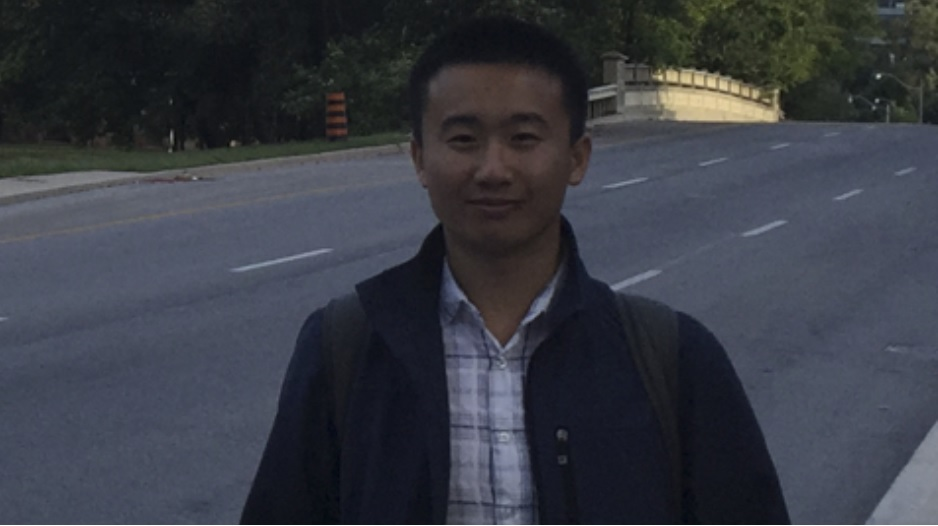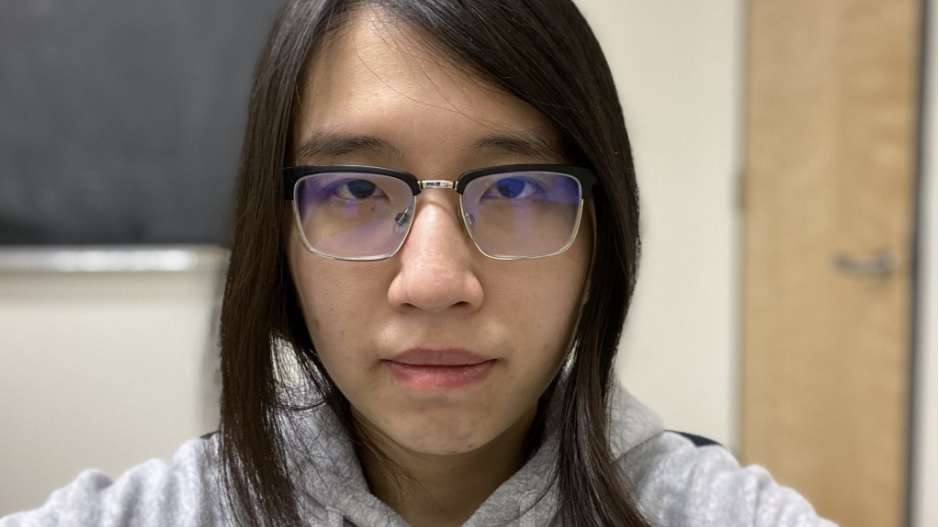## Welcome to The Computational Mathematics Group at the University of Alberta!Computational methods are extraordinarily useful in helping us to understand mathematical models arising from many physical phenomena. Our group consists of faculty members and graduate students at the University of Alberta's Department of Mathematical and Statistical Sciences. We are interested in using insights from mathematical analysis to develop efficient numerical algorithms for solving problems in science and engineering such as high-frequency wave propagation, seismic imaging, fluid dynamics, aerodynamics, etc. We employ and develop various tools and methods in applied mathematics such as wavelets/framelet methods, finite difference method, finite element method, neural networks, and parallel computing to tackle problems of interest. Over the years, our research work has been partly supported by Natural Sciences and Engineering Research Council of Canada (NSERC), Westgrid, Compute Canada Calcul Canada, and MITACS.

Department of Mathematical and Statistical Sciences
University of Alberta
Edmonton, Alberta

## Faculty MembersBin Han,  Professor of Mathematics Email: bhan_AT_ualberta.ca   Google Scholar   MathSciNet Research interests: Applied harmonic analysis: Wavelet theory with applications to image processing, data sciences, and numerical PDEs, spline and sparse approximation Computational mathematics and scientific computing: Time-harmonic wave propagation problems, the Helmholtz equation, Burgers' equations, PDEs with rough coefficients and singularity, interface problems Computer Aided Geometric Design (CAGD): Subdivision schemes for curves and surfaces, spline approximation Deep learning and data sciencesPeter Minev,  Professor of Mathematics Email: minev_AT_ualberta.ca   Google Scholar   MathSciNet Research interests: Fictitious domain methods for fluid-structure interaction problems Methods for free boundary problems Lagrangian and Eulerian-Lagrangian algorithms using finite elements Projection and artificial compressibility methods for the incompressible Navier-Stokes equations Numerical methods for MHD Mathematical modelling of multiphase flows Scientific computing/supercomputing; parallel algorithmsYau Shu Wong,  Professor of Mathematics Email: yauwong_AT_ualberta.ca   ResearchGate   MathSciNet Research interests: Applied and industrial mathematics Numerical analysis and scientific computing Solving practical problems in sciences and engineering                 Computational aerodynamics and aeroelasticity Nonlinear dynamical systems Data mining techniquesQiwei Feng,  PhD student Email: qfeng_AT_ualberta.ca  arXiv   MathSciNet Qiwei is a PhD student supervised by Dr. Peter Minev and Dr. Bin Han. Major awards and scholarships: Alberta Graduate Excellence Scholarship, Chu Shu Ying Graduate Scholarship in Computational Mathematics. Research interests: High order finite difference methods for Poisson interface problems with singular sources Discrete maximum principle for elliptic interface problems with discontinuous coefficients Helmholtz interface problems with reduced pollution. High frequency solutions in the porous media equations, and singular perturbation problems. Publications during graduate program: Q. Feng, B. Han, and P. Minev, Sixth order compact finite difference schemes for Poisson interface problems with singular sources, Computers & Mathematics with Applications, Vol. 99 (2021), 2-25.Michelle Michelle,  PhD student Email: mmichelle_AT_ualberta.ca   ResearchGate   MathSciNet Michelle Michelle is a PhD student supervised by Dr. Bin Han and Dr. Yau Shu Wong. Major awards and scholarships: The Josephine Mitchell Research Prize, NSERC CSGD and CGSM, Alberta Innovates Graduate Scholarship, President's Doctoral Prize of Distinction, Alberta Graduate Excellence Scholarship, Chu Shu Ying Graduate Scholarship in Computational Mathematics. Research interests: Wavelet theory and methods for numerical PDEs Finite difference methods for numerical PDEs including the Helmholtz equation and wave propagation models. Stability analysis for the Helmholtz equation Publications during graduate program: B. Han and M. Michelle, Wavelets on intervals derived from arbitrary compactly supported biorthogonal multiwavelets, Applied and Computational Harmonic Analysis, Vol. 53 (2021), 270-331. B. Han, M. Michelle and Y. S. Wong, Dirac assisted tree method for 1D Helmholtz equations with arbitrary variable wave numbers, Computers & Mathematics with Applications, Vol. 97 (2021), 416-438. B. Han and M. Michelle, Derivative-orthogonal Riesz wavelets in Sobolev spaces with applications to differential equations, Applied and Computational Harmonic Analysis, Vol. 47 (2019), Issue 3, 759-794. B. Han and M. Michelle, Construction of wavelets and framelets on a bounded interval, Analysis and Applications, Vol. 16 (2018), 807-849.

## Recent alumniRan Lu,  PhD (2021) Email: rlu3_AT_ualberta.ca   ResearchGate   MathSciNet Dr. Ran Lu is currently an assistant professor at Hohai University. Dr. Lu completed his PhD under the supervision of Dr. Bin Han. The title of his PhD thesis is Several studies on framelets derived from compactly supported refinable vector functions. In his PhD thesis, he has studied both univariate and multivariate quasi-tight framelets derived from refinable vector functions as well as several other topics in wavelet analysis. Publications during graduate program: B. Han and R. Lu, Multivariate quasi-tight framelets with high balancing orders derived from any compactly supported refinable vector functions, Science China Mathematics, Vol. 65 (2022), 81-110. B. Han and R. Lu, Compactly supported quasi-tight multiframelets with high balancing orders and compact framelet transforms, Vol. 51 (2021), 295-332. Applied and Computational Harmonic Analysis, R. Lu, On the strong restricted isometry property of Bernoulli random matrices, Journal Approximation Theory, Vol. 245 (2019), 1-22.Rahim Usubov,  MSc (2021) Email: usubov_AT_ualberta.ca   Mr. Usubov completed his MSc under the supervision of Dr. Peter Minev. Publications during graduate program:Roman Frolov,  PhD (2020) Email: frolov_AT_ualberta.ca   Dr. Roman Frolov is currently working in the industry. Dr. Frolov completed his PhD under the supervision of Dr. Peter Minev. The title of his PhD thesis is Splitting schemes for compressible Navier-Stokes equations. Publications during graduate program: A. Takhirov, R. Frolov, and P. Minev, A direction splitting scheme for Navier–Stokes–Boussinesq system in spherical shell geometries, International Journal for Numerical Methods in Fluids, Vol. 93 (2021), 3507-3523. R. Frolov, P. Minev, and A. Takhirov, An efficient algorithm for weakly compressible flows in spherical geometries, International Journal for Numerical Methods in Fluids, Vol. 93 (2021), 1359-1377. R. Frolov, An efficient algorithm for the multicomponent compressible Navier–Stokes equations in low-and high-Mach number regimes, Computers & Fluids, Vol. 178 (2019), 15-40.Chenzhe Diao,  PhD (2018) Email: diao_AT_ualberta.ca   Dr. Chenzhe Diao is currently working in the industry. Dr. Diao completed his PhD under the supervision of Dr. Bin Han. The title of his PhD thesis is Spectral Factorization of Matrices of Laurent Polynomials and Construction of Quasi-tight Framelets. Publications during graduate program: C. Diao and B. Han, Quasi-tight framelets with high vanishing moments derived from arbitrary refinable functions, Applied and Computational Harmonic Analysis, Vol. 49 (2020), 123-151. C. Diao and B. Han, Generalized matrix spectral factorization and quasi-tight framelets with a minimum number of generators, Mathematics of Computation, Vol. 89 (2020), 2867-2911.

## Some journals on computational mathematics and scientific computing

• Applied and Computational Harmonic Analysis
• Applied Mathematics and Computation
• Applied Numerical Mathematics
• Communications in Computational Physics
• Computers & Mathematics with Applications
• Foundations of Computational Mathematics
• IMA Journal of Numerical Analysis
• International Journal of Numerical Analysis & Modeling
• Journal of Computational and Applied Mathematics
• Journal of Computational Physics
• Journal of Scientific Computing
• Mathematics of Computation
• Multiscale Modeling and Simulation (SIAM)
• Numerical Algorithms
• Numerische Mathematik
• SIAM Journal on Imaging Sciences
• SIAM Journal on Mathematical Analysis
• SIAM Journal on Numerical Analysis
• SIAM Journal on Scientific Computing